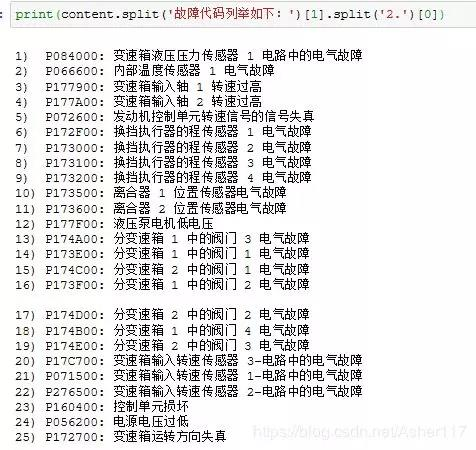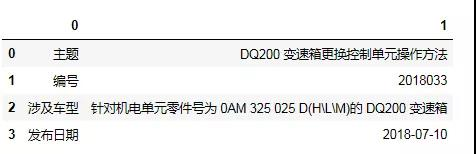# Python 解析 PDF 文本和表格的四大方法介绍

## 1. PyPDF2 解析 PDF 文档

Python for NLP: Working with Text and PDF Files

``````pip install PyPDF2
#---------OR
conda install -c conda-forge pypdf2
``````

``````import PyPDF2
path = r"****.pdf"
#使用open的‘rb’方法打开pdf文件（这里必须得使用二进制rb的读取方式）
mypdf = open(path,mode='rb')
#调用PdfFileReader函数
pdf_document = PyPDF2.PdfFileReader(mypdf)
#使用pdf_document变量，获取各个信息
#或者PDF文档的页数
pdf_document.numPages
#输出PDF文档的第一页内容
first_page = pdf_document.getPage(0)
print(first_page.extractText())
``````

## 2. pdfplumber 解析 PDF 文档

``````pip install pdfplumber
``````

（1）解析文本内容
pdfplumber 中的 extract_text 函数是可以直接识别 PDF 中的文本内容。

``````import pdfplumber
import pandas as pd
with pdfplumber.open(path) as pdf:
content = ''
#len(pdf.pages)为PDF文档页数
for i in range(len(pdf.pages)):
#pdf.pages[i] 是读取PDF文档第i+1页
page = pdf.pages[i]
#page.extract_text()函数即读取文本内容，下面这步是去掉文档最下面的页码
page_content = '\n'.join(page.extract_text().split('\n')[:-1])
content = content + page_content
print(content)
````````````print(content.split('故障代码列举如下：').split('2.'))
``````（2）解析表格内容

``````import pdfplumber
import pandas as pd

with pdfplumber.open(path) as pdf:
first_page = pdf.pages
for table in first_page.extract_tables():
df = pd.DataFrame(table)
df
``````## 3. pdfminer3k 解析 PDF 文档

pdfminer3k 是 pdfminer 的 python3 版本，主要用于读取 pdf 中的文本。如果直接搜索 pdfminer3k 的话会发现网上有非常多的教程，但是看了之后，你可能就想吐槽这些教程太繁琐了，看着头疼。pdfminer 方法解析 PDF 可以很好的提取文本内容，但是对于表格数据，能提取出文字，但是没有格式，会很不友好。因此你如果只需要提取文本内容的话，可以使用 pdfminer 扩展包，这个包也能很好的支持中文。

## 4. Camelot 解析 PDF 文档

Camelot 先使用 pip install camelot-py 语句安装，如果报错，参考安装 Camelot 教程。

``````import camelot
import pandas as pd
tables = camelot.read_pdf(filepath=path,pages='1',flavor='stream')
df = pd.DataFrame(tables.data)
``````

Camelot 读取 PDF 文件中的表格数据很好用，并且能够很好的支持中文，但是 Camelot 有很多局限性。